In an experiment of find the focal length of a concave mirror a graph is drawn between the magnitudes of u and v.  The graph looks like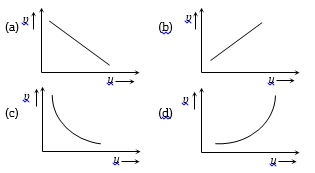Concept Questions :-

Reflection at spherical surface
High Yielding Test Series + Question Bank - NEET 2020

Difficulty Level:

As the position of an object (u) reflected from a concave mirror is varied, the position of the image (v) also varies. By letting the u changes from 0 to  the graph between v versus u will be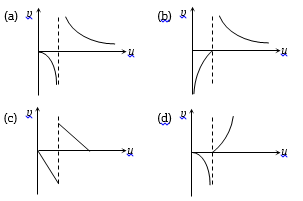Concept Questions :-

Reflection at spherical surface
High Yielding Test Series + Question Bank - NEET 2020

Difficulty Level:

The graph between u and v for a convex mirror is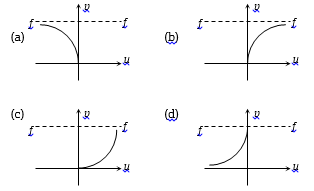Concept Questions :-

Reflection at spherical surface
High Yielding Test Series + Question Bank - NEET 2020

Difficulty Level:

A glass prism $\left(\mu =1.5\right)$ is dipped in water $\left(\mu =4/3\right)$ as shown in figure. A light ray is incident normally on the surface AB. It reaches the surface BC after totally reflected, if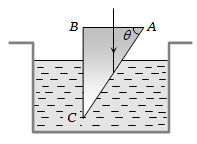(a)                             (b)

(c)                             (d) It is not possible

Concept Questions :-

Total internal reflection
High Yielding Test Series + Question Bank - NEET 2020

Difficulty Level:

A convex lens A of focal length 20 cm and a concave lens B of focal length 5 cm are kept along the same axis with the distance d between them. If a parallel beam of light falling on A leaves B as a parallel beam, then distance d in cm will be

(a) 25                         (b) 15

(c) 30                          (d) 50

Concept Questions :-

Lenses
High Yielding Test Series + Question Bank - NEET 2020

Difficulty Level:

A medium shows relation between i and r as shown. If speed of light in the medium is nc then value of n is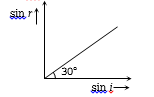(a)   1.5

(b)   2

(c)   2–1

(d)   3–1/2

Concept Questions :-

Refraction at plane surface
High Yielding Test Series + Question Bank - NEET 2020

Difficulty Level:

For a concave mirror, if the virtual image is formed, the graph between m and u is of the form :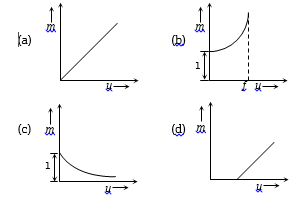Concept Questions :-

Reflection at spherical surface
High Yielding Test Series + Question Bank - NEET 2020

Difficulty Level:

For a convex lens, the distance of the object is taken on X-axis and the distance of the image is taken on Y-axis, the nature of the graph so obtained is :

(a) Straight line                      (b) Circle

(c) Parabola                           (d) Hyperbola

Concept Questions :-

Lenses

Difficulty Level:

The diameter of a plano-convex lens is 6 cm and thickness at the centre is 3 mm. If the speed of light in the material of the lens is $2×{10}^{8}m}{sec}$, the focal length of the lens is

(a) 15 cm                         (b) 20 cm

(c) 30 cm                         (d) 10 cm

Concept Questions :-

Lenses
High Yielding Test Series + Question Bank - NEET 2020

Difficulty Level:

A luminous object is placed at a distance of 30 cm from the convex lens of focal length 20 cm. On the other side of the lens, at what distance from the lens, a convex mirror of radius of curvature 10 cm be placed in order to have an upright image of the object coincident with it?

(a) 12 cm                              (b) 30 cm

(c) 50 cm                              (d) 60 cm

Concept Questions :-

Lenses